# Modified internal rate of return

The modified internal rate of return (MIRR) is a financial measure of an investment's attractiveness. It is used in capital budgeting to rank alternative investments of equal size. As the name implies, MIRR is a modification of the internal rate of return (IRR) and as such aims to resolve some problems with the IRR.

## Problems with the IRR

While there are several problems with the IRR, MIRR resolves two of them.

Firstly, IRR is sometimes misapplied, under an assumption that interim positive cash flows are reinvested at the same rate of return as that of the project that generated them. This is usually an unrealistic scenario and a more likely situation is that the funds will be reinvested at a rate closer to the firm's cost of capital. The IRR therefore often gives an unduly optimistic picture of the projects under study. Generally for comparing projects more fairly, the weighted average cost of capital should be used for reinvesting the interim cash flows.

Secondly, more than one IRR can be found for projects with alternating positive and negative cash flows, which leads to confusion and ambiguity. MIRR finds only one value.

## Calculation of the MIRR

MIRR is calculated as follows: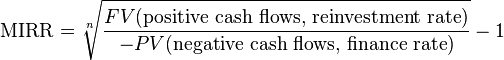$\mbox{MIRR}=\sqrt[n]{\frac{FV(\text{positive cash flows, reinvestment rate})}{-PV(\text{negative cash flows, finance rate})}}-1$,

where n is the number of equal periods at the end of which the cash flows occur (not the number of cash flows), PV is present value (at the beginning of the first period), FV is future value (at the end of the last period).

The formula adds up the negative cash flows after discounting them to time zero using the external cost of capital, adds up the positive cash flows including the proceeds of reinvestment at the external reinvestment rate to the final period, and then works out what rate of return would cause the magnitude of the discounted negative cash flows at time zero to be equivalent to the future value of the positive cash flows at the final time period.

Spreadsheet applications, such as Microsoft Excel, have inbuilt functions to calculate the MIRR. In Microsoft Excel this function is "=MIRR".

### Example

If an investment project is described by the sequence of cash flows:

Year Cash flow
0 -1000
1 -4000
2 5000
3 2000

then the IRR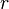$r$ is given by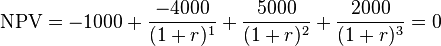$\mbox{NPV} = -1000 + \frac{-4000}{(1+r)^1} + \frac{5000}{(1+r)^2} + \frac{2000}{(1+r)^3} = 0$.

In this case, the answer is 25.48% (with this conventional pattern of cash flows, the project has a unique IRR).

To calculate the MIRR, we will assume a finance rate of 10% and a reinvestment rate of 12%. First, we calculate the present value of the negative cash flows (discounted at the finance rate):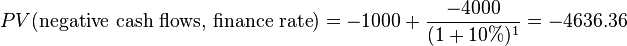$PV(\text{negative cash flows, finance rate}) = -1000 + \frac{-4000}{(1+10\%)^1} = -4636.36$.

Second, we calculate the future value of the positive cash flows (reinvested at the reinvestment rate):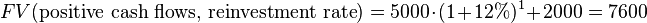$FV(\text{positive cash flows, reinvestment rate}) = 5000\cdot(1+12\%)^1 + 2000 = 7600$.

Third, we find the MIRR: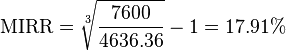$\mbox{MIRR}=\sqrt{\frac{7600}{4636.36}}-1=17.91\%$.

The calculated MIRR (17.91%) is significantly different from the IRR (25.48%).

## Comparing projects of different sizes

Like the internal rate of return, the modified internal rate of return is not valid for ranking projects of different sizes, because a larger project with a smaller modified internal rate of return may have a higher net present value. However, there exist variants of the modified internal rate of return which can be used for such comparisons.

## References

1. Lin, S. A. Y., "The modified internal rate of return and investment criterion," The Engineering Economist 21(4), 1976, 237-247.
2. Beaves, R. G., "Net present value and the rate of return: Implicit and explicit reinvestment assumptions," The Engineering Economist 33, 1988, 275-302.
3. Internal Rate of Return: A Cautionary Tale
4. Shull, D. M., "Efficient capital project selection through a yield-based capital budgeting technique," The Engineering Economist 38(1), 1992, 1-18.
5. Hajdasinski, M., "Remarks in the context of 'The case for the generalized net present value formula'," The Engineering Economist 40(2), 1995, 201-210.